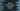# 3 ways in Python to find the middle n list elements## Python program to find the middle n elements of a list:

In this post, we will learn how to find and print the middle n elements of a list in Python. The program will use a predefined list and take the value of n from the user.

We will also learn how to take the list numbers as inputs from the user to find the middle n elements. I will show you different ways to solve this problem.

### Algorithm:

Let’s consider the following list:

``[1, 2, 3, 4, 5, 6, 7, 8]``

The middle 4 elements of this list are `3, 4, 5`, and `6`. To find these elements, we have to find the start and end indices of the list. We can use the following steps to find the start and the end indices:

1. Suppose n is the number of middle elements we want to find.
2. Find the length of the list. Divide this by 2.
3. Divide the n value by 2.
4. Subtract the value of step 3 from the value of step 2 to get the start index of the middle elements sublist.
5. Extract n elements from the start index to get the required middle elements.

We can use list slicing or iterate over the indices to pick the middle elements.

### Example 1: Python program to find the middle n elements of a list by using a loop:

In this example, the program will use a for loop to iterate over the indices to find the mid n elements of the list.

``````given_list = [1, 2, 3, 4, 5, 6, 7, 8, 9]
n = 3

list_len = len(given_list)
start = list_len//2 - n//2

result_list = []

for i in range(start, start+n):
result_list.append(given_list[i])

print(f'Given list: {given_list}')
print(f'Middle {n} elements of the list: {result_list}')``````

Here,

• given_list is the given list of numbers.
• The variable n represents the number of middle elements to find.
• It uses the len() method to find the length of the list and assigns it to the list_len variable.
• The starting index of the middle element list is assigned to the variable start.
• It uses a for loop to find the n elements starting from the start index. The result elements are appended to the list result_list.
• The last two lines are printing the given list and the result list.

If you run this program, it will print the below output:

``````Given list: [1, 2, 3, 4, 5, 6, 7, 8, 9]
Middle 3 elements of the list: [4, 5, 6]``````

### Example 2: Python program to find the middle n elements of a list by using list slicing:

We can also use list slicing to find the middle n elements of a list. If we want to get the elements between indices start and end, we can use the below list slicing syntax:

``list[start:end+1]``

It will be similar to the above example. It will find the starting index of the middle element list and with list slicing, we can find the n elements starting from that index.

``````given_list = [1, 2, 3, 4, 5, 6, 7, 8]
n = 4

list_len = len(given_list)
start = list_len//2 - n//2

result_list = given_list[start:start+n]

print(f'Given list: {given_list}')
print(f'Middle {n} elements of the list: {result_list}')``````

This is similar to the above example. But instead of a for loop, we are using list slicing to find the middle elements.

If you run this program, it will print the below output:

``````Given list: [1, 2, 3, 4, 5, 6, 7, 8]
Middle 4 elements of the list: [3, 4, 5, 6]``````

### Example 3: By using user input values:

The following example uses user input values to create the list. It also reads the value of n as input.

``````given_list = []

list_len = int(input("Enter the length of the list: "))
for i in range(list_len):
given_list.append(int(input(f'Enter the number for index {i}: ')))

n = int(input("Enter the value of n: "))

list_len = len(given_list)
start = list_len//2 - n//2

result_list = given_list[start:start+n]

print(f'Given list: {given_list}')
print(f'Middle {n} elements of the list: {result_list}')``````

In this example, it reads the list values as inputs from the user and creates the list.

• The list_len variable is used to hold the length of the list.
• By using a for loop, it reads the numbers as inputs from the user.
• It also reads the value of n from the user.

It will give output as below:

``````Enter the length of the list: 5
Enter the number for index 0: 1
Enter the number for index 1: 2
Enter the number for index 2: 3
Enter the number for index 3: 4
Enter the number for index 4: 5
Enter the value of n: 3
Given list: [1, 2, 3, 4, 5]
Middle 3 elements of the list: [2, 3, 4]``````